## Thursday, July 17, 2014

### Alternate Price Plots | ggplot2 + magrittr

Just some quick experiments with ggplot2 + magrittr to plot prices differently than the traditional ways. We wi€™ll get daily data on the S&P 500 from FRED using `getSymbols` and then push it through a `magrittr` pipe to various alternative plots.

A couple notes for those who might be interested in magrittr. If you want to `->` or `<-` but do not want the pipe fun to end, then you can use `assign` like this.

``mydata  %T>% assign( x="sp_df", value = ., envir = .GlobalEnv ) %>%``

Also for those who want to use the special `+` inside a pipe or just want your `+` on a different line for tidy code and easy commenting, then you can do like this.

``ggplot( ) %>%  + geom_point()``

Let me know if I have it all wrong, and now some plots as promised.

``require(ggplot2)require(dplyr)require(magrittr)require(quantmod)getSymbols("SP500", src="FRED", from = "1900-01-01", auto.assign=F) %>%  na.omit %>%  data.frame(    date = index(.)  ) %T>% assign( x="sp_df", value = ., envir = .GlobalEnv ) %>%  mutate( year = format(date,"%Y") ) %>%  ggplot(  aes( x=SP500, group = year ) ) %>%    + geom_density() %>%    + facet_wrap(~year,nrow=1) %>%     + coord_flip() %>%    + theme_bw() %>%    + theme(        axis.line=element_blank()        ,axis.text.x=element_blank()        ,axis.title.x=element_blank()      ) ``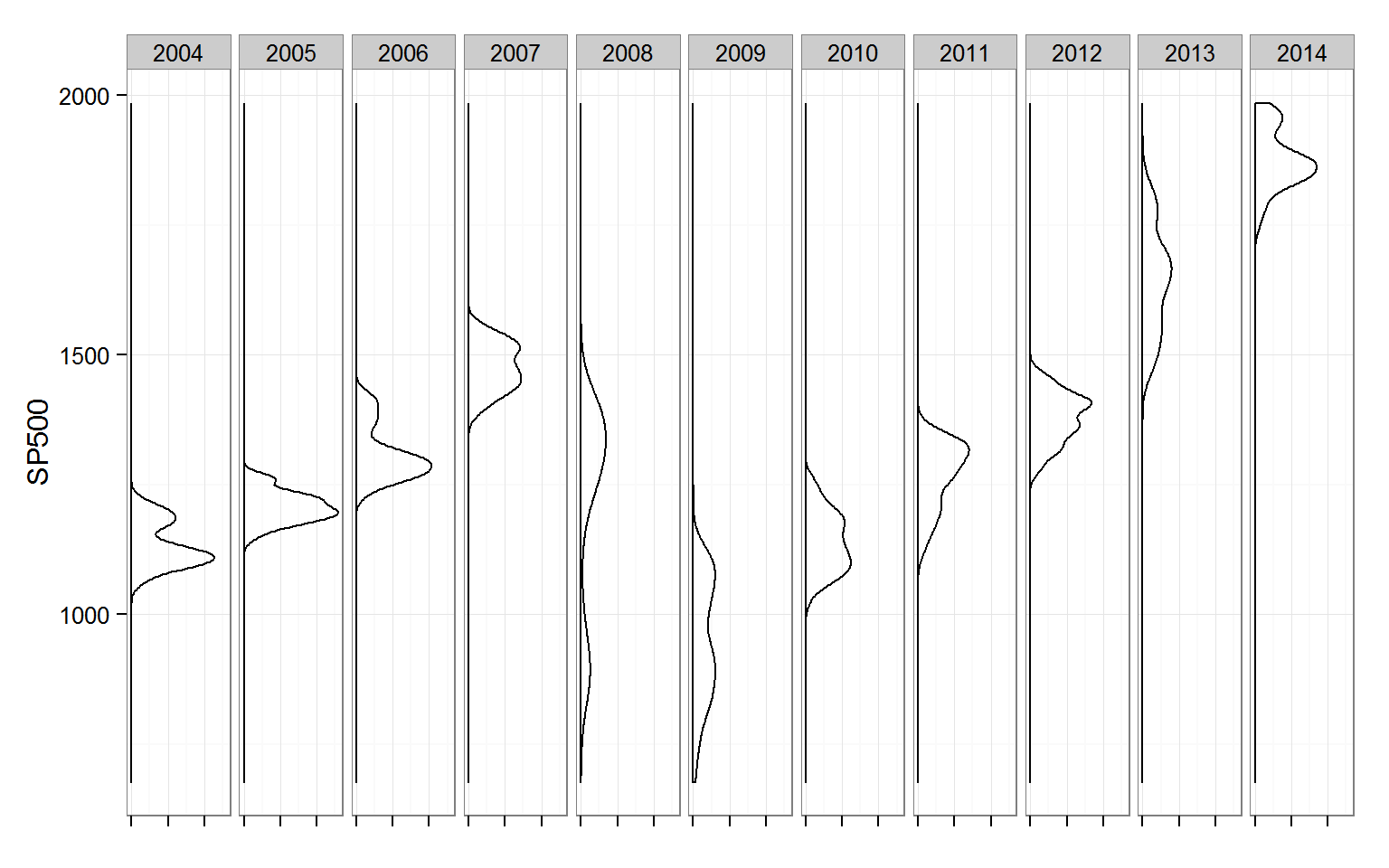``sp_df %>%  ggplot( aes( x = format(date,"%Y"), y = SP500 ) ) %T>%   ( function(x){ print( x + geom_line() ) } ) %T>%  ( function(x){ print( x + geom_point() ) } ) %T>%  ( function(x){ print( x + geom_hex( bins = 20 ) ) } ) %>%  + geom_violin()``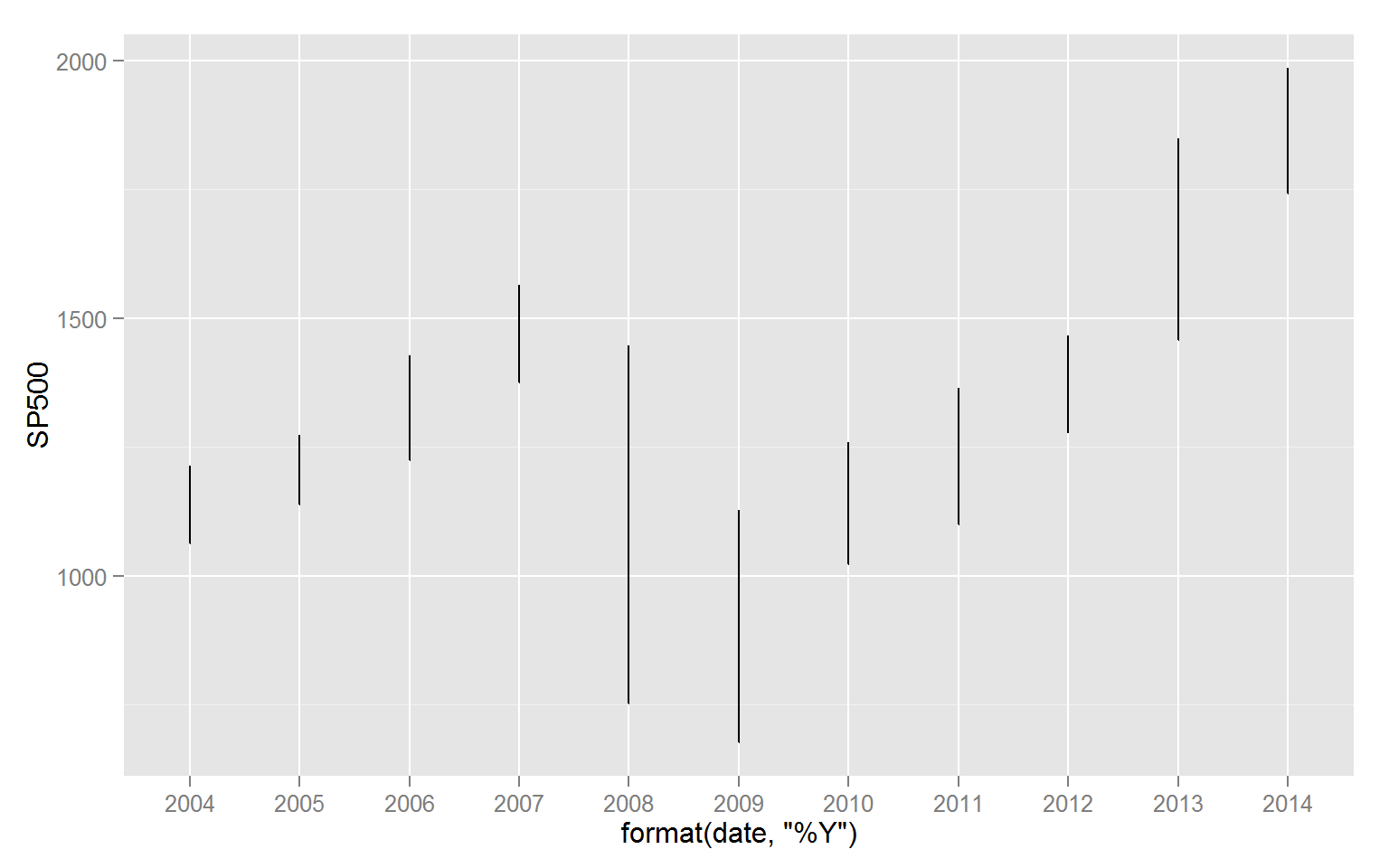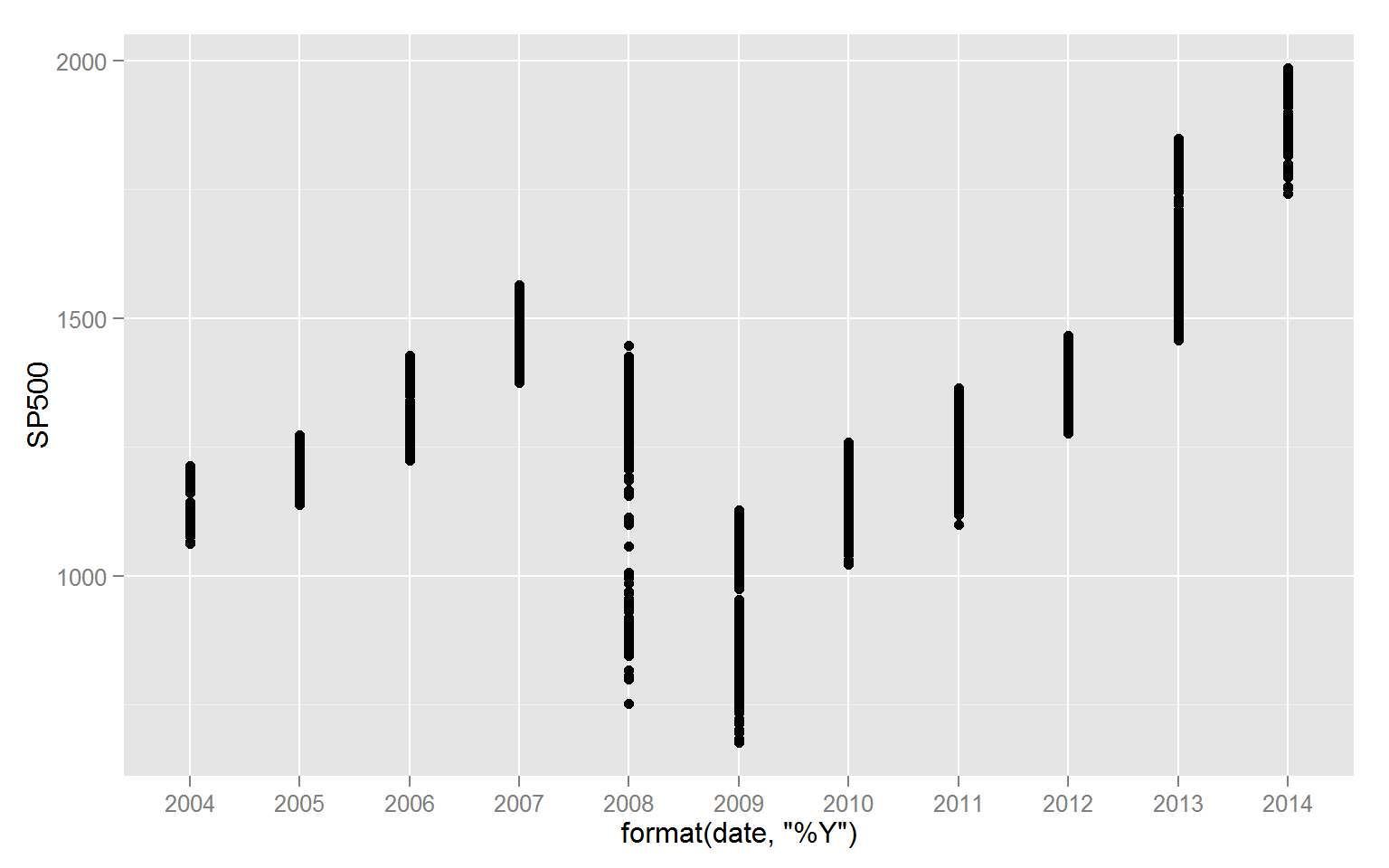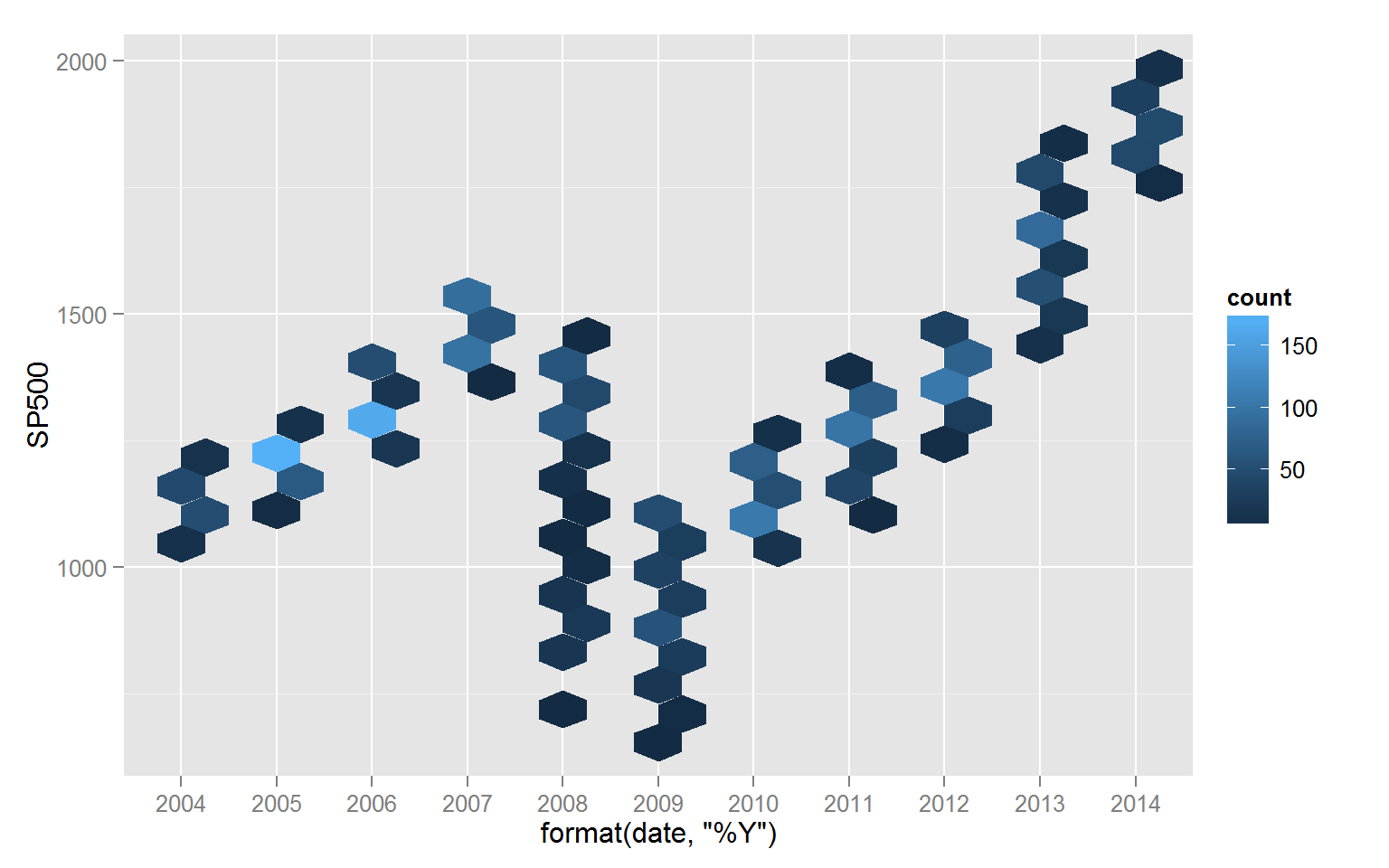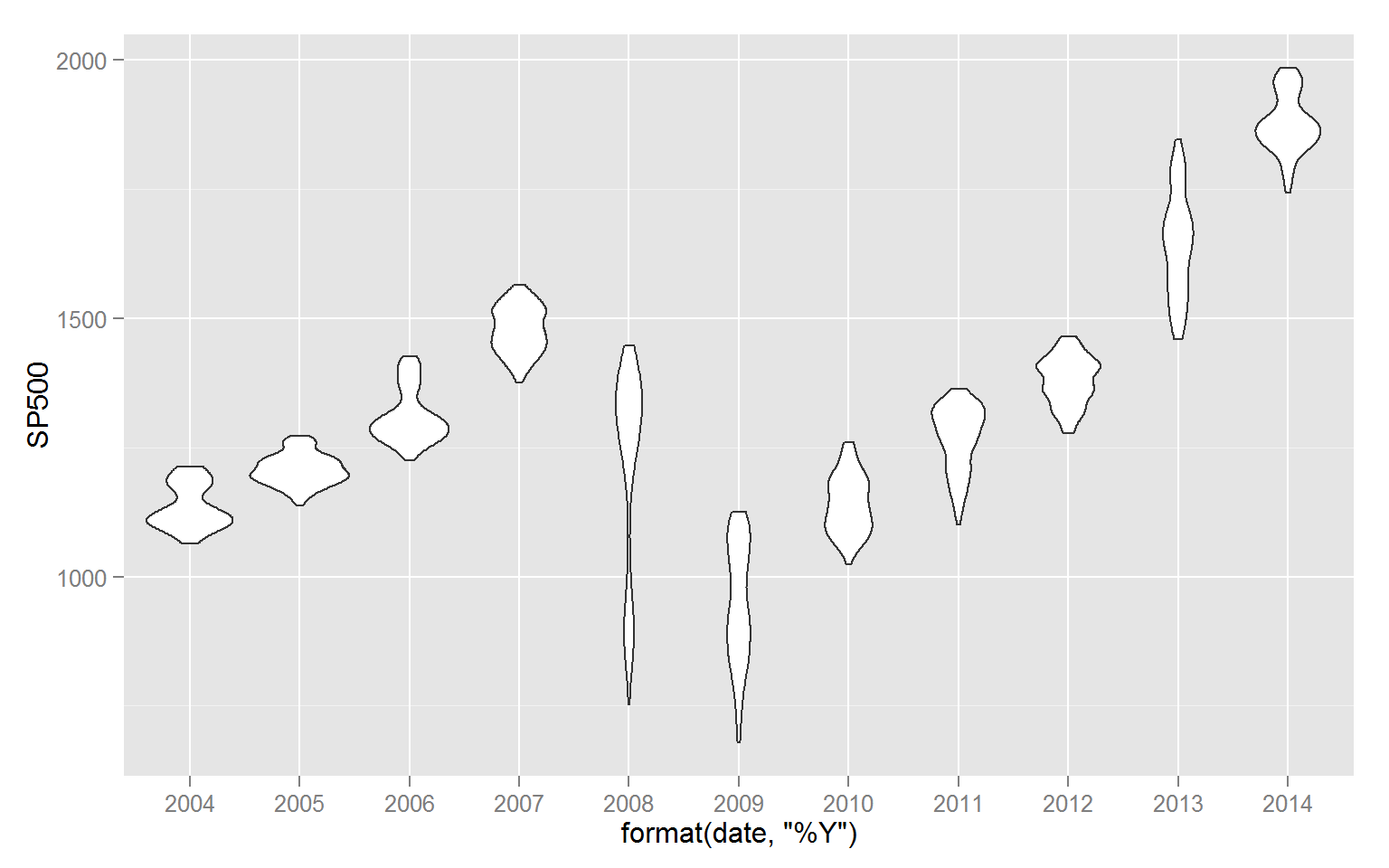If you are interested in seeing the source Rmd, then it is here. One more note is this is copied/pasted with only minor changes from Rstudio knitted Rmd.

1.Is the T meant to be in "%T>%"? I have never seen that before and your code won't run on my machine either way (with or without the T)

2.The error I get it "Error in index(.) : object '.' not found"

3.you'll need to get the newest magrittr with

devtools::install_github("smbache/magrittr")

If you have dplyr, you'll need to require or library(magrittr) last to make sure %>% is from magrittr. Also, it might be helfpul if you read the Readme.md from magrittr on its github page https://github.com/smbache/magrittr.

4.Bizarre, %T>% still doesn't work even after updating the package but I can get the chart to work without it, thanks!